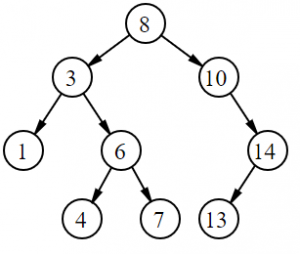# Binary Trees: Definition, Examples

Share onBinary Trees are graphs or tree data structures where each node (shown as circles in the graph to the left) has up to a possible two branches (‘children’). These are called the left branch and right branch, or, sometimes, the left child and right child.

Although they are relatively simple structures, binary trees are extremely useful for modeling data. For example, rational numbers can be represented with a variant of the binary tree, called the Calkin–Wilf tree. They can also be used to represent hierarchies, and reflect structural relationships in data. They are flexible; we can move ‘subtrees’ around easily within a tree as needed. They can also be very efficiently searched and analyzed by computer.

## Important Definitions for Binary Trees

The top node of a tree (8 in the above image) is called the root node.

An external node is one without child branches, while an internal node has at least one child branch.

The size of a binary tree refers to the number of nodes it has.

The distance from a node B to the root node is the level of B. The root is at level 0, the root’s children are at level 1, and so on.

The sum of the levels of each of the internal nodes in a tree is called the internal path length of that tree.

The sum of the levels of each of the external nodes in a tree is called the external path length.

The maximum level, among all of the external nodes, is called the height of the tree.

## More Terminology of Binary Trees

A tree that has a root node is called a rooted tree. If every node has at most two branches, it becomes a binary tree and is a rooted binary tree.

A tree where every node (except for the leaves) has 2 branches is called a full binary tree. Full binary trees are sometimes also called proper or plane binary trees.

A tree where all interior nodes have two branches and all nodes with 0 branches (leaf nodes) are at the same level or have the same depth is called a perfect binary tree.

A binary tree which is completely filled—except potentially the bottom level, which is filled from left to right—is called a complete binary tree. Complete binary trees are important because they provide the best ratio between a tree’s height and the number of nodes.

## References

Sedgewick & Flajolet. An Introduction to the Analysis of Algorithms. Retrieved November 24 2017 from: